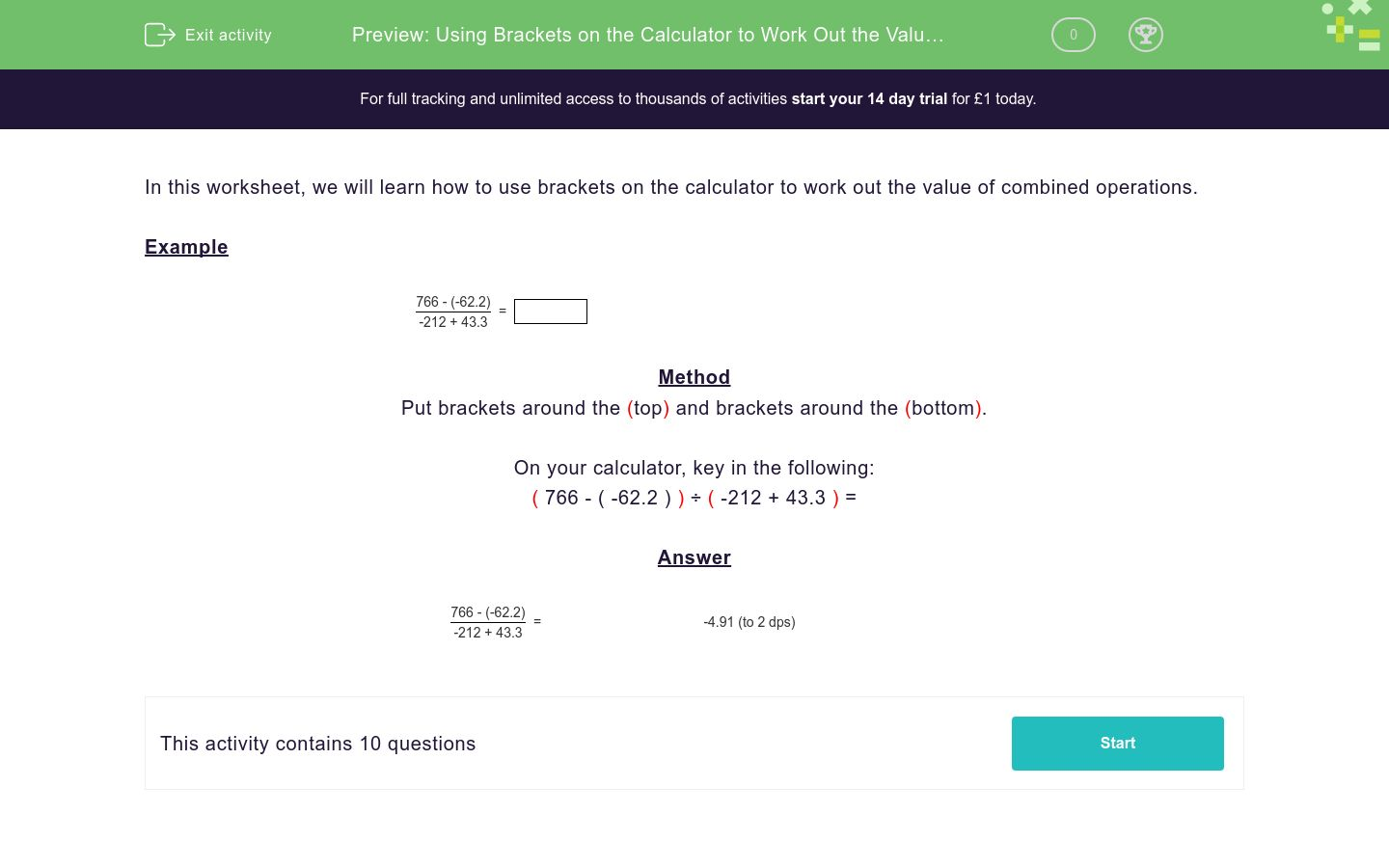# Using Brackets on the Calculator to Work Out the Value of Combined Operations

In this worksheet, students use brackets on the calculator to work out the value of combined operations.Key stage:  KS 3

Curriculum topic:   Number

Curriculum subtopic:   Use Calculators/Technology for Accuracy

Difficulty level:### QUESTION 1 of 10

In this worksheet, we will learn how to use brackets on the calculator to work out the value of combined operations.

Example

 766 - (-62.2) -212 + 43.3
=

Method

Put brackets around the (top) and brackets around the (bottom).

On your calculator, key in the following:

( 766 - ( -62.2 ) ) ÷ ( -212 + 43.3 ) =

 766 - (-62.2) -212 + 43.3
=       -4.91 (to 2 dps)

Work out:

78.4 - (50.2 + 8)2   =

Work out:

75 - (60.1 + 6)2   =

Work out:

 -38 × (-7.81)2 5.07 + 37
=

Work out:

 436 - (-17.8) -101 + 10.7
=

Work out:

21.2 - (70.2 + 3)2   =

Work out:

 -59 × (-8.31)2 7.21 + 96
=

Calculate:

 227 - (-35.1) -932 + 81.3
=

Calculate:

 -45 × (-5.15)2 -8.51 - 51
=

Work out:

 235 - (-29.1) -463 + 26
=

Work out:

 232 - (-2.1) -46 + 2.6
=

• Question 1

Work out:

78.4 - (50.2 + 8)2   =
-3308.84
EDDIE SAYS
You need to put one extra set of brackets here to show that you have to square before you subtract

78.4 - ((50.2 + 8)2)

• Question 2

Work out:

75 - (60.1 + 6)2   =
-4294.21
EDDIE SAYS
You need to put one extra set of brackets here to show that you have to square before you subtract

75 - ((60.1 + 6)2)

• Question 3

Work out:

 -38 × (-7.81)2 5.07 + 37
=

-55.1
EDDIE SAYS
Don't forget you need to divide all of the top by all of the bottom. You need to put brackets around the entire numerator and the entire denominator
• Question 4

Work out:

 436 - (-17.8) -101 + 10.7
=

-5.03
EDDIE SAYS
Don't forget you need to divide all of the top by all of the bottom. You need to put brackets around the entire numerator and the entire denominator
• Question 5

Work out:

21.2 - (70.2 + 3)2   =
-5337.04
EDDIE SAYS
You need to put one extra set of brackets here to show that you have to square before you subtract

21.2 - ((70.2 + 3)2)

• Question 6

Work out:

 -59 × (-8.31)2 7.21 + 96
=

-39.48
EDDIE SAYS
Don't forget you need to divide all of the top by all of the bottom. You need to put brackets around the entire numerator and the entire denominator
• Question 7

Calculate:

 227 - (-35.1) -932 + 81.3
=

-0.31
EDDIE SAYS
Don't forget you need to divide all of the top by all of the bottom. You need to put brackets around the entire numerator and the entire denominator
• Question 8

Calculate:

 -45 × (-5.15)2 -8.51 - 51
=

20.06
EDDIE SAYS
Don't forget you need to divide all of the top by all of the bottom. You need to put brackets around the entire numerator and the entire denominator
• Question 9

Work out:

 235 - (-29.1) -463 + 26
=

-0.6
EDDIE SAYS
Don't forget you need to divide all of the top by all of the bottom. You need to put brackets around the entire numerator and the entire denominator
• Question 10

Work out:

 232 - (-2.1) -46 + 2.6
=

-12.2
EDDIE SAYS
Don't forget you need to divide all of the top by all of the bottom. You need to put brackets around the entire numerator and the entire denominator
---- OR ----

Sign up for a £1 trial so you can track and measure your child's progress on this activity.

### What is EdPlace?

We're your National Curriculum aligned online education content provider helping each child succeed in English, maths and science from year 1 to GCSE. With an EdPlace account you’ll be able to track and measure progress, helping each child achieve their best. We build confidence and attainment by personalising each child’s learning at a level that suits them.

Get started